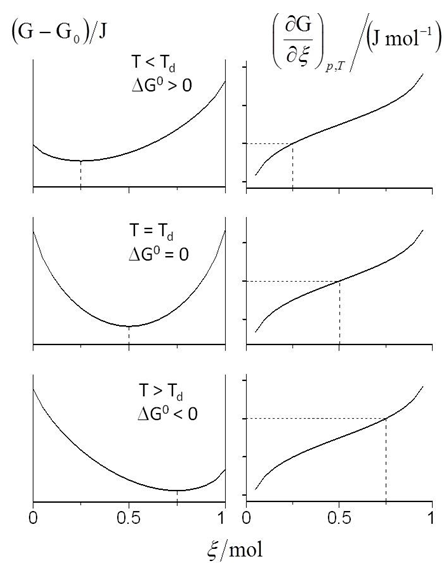## Figure 4. Gibbs free-energy of protein denaturation (G-G0, left) and derivative (∂G/∂ξ)T,p, (right) as a function of the degree of advancement ξ at the denaturation temperature Td (middle), below (upper) and above (lower) Td according to eqs. (5) and (6), respectively. The calculation refers to the α-Chymotrypsinogen A denaturation with Td = (273+55) K, Tbelow = (Td – 2) K, Tupper = (Td + 2) K and with the equilibrium constant values determined according to the Results Section. For the sake of simplicity n0 has been taken equal to 1 mol. The equilibrium state is specified by the (G-G0) minimum on the left and by the zero value of the (∂G/∂ξ)T,p derivative (the horizontal dashed lines) on the right. The equilibrium composition of the D form is indicated in correspondence on the two abscissa scales

#### Laura Moroni, Cristina Gellini, Pier Remigio Salvi

World Journal of Chemical Education. 2015, 3(3), 59-63 doi:10.12691/wjce-3-3-1